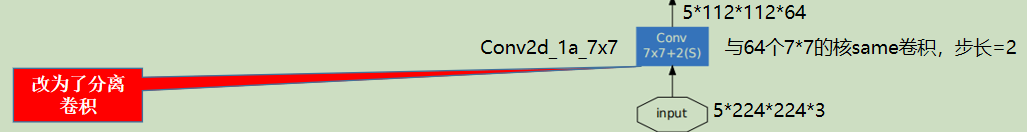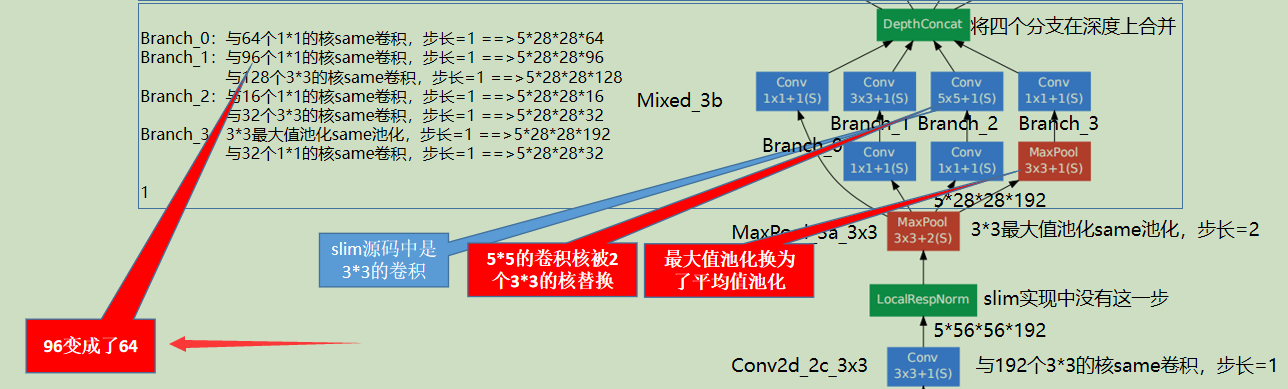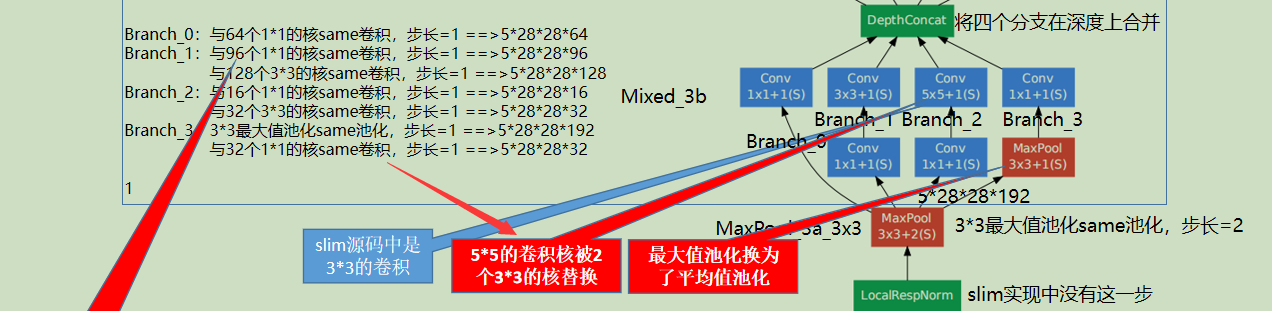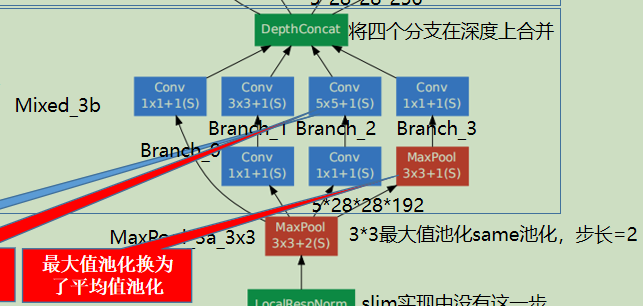# 目录

## 参考资料

 第一层卷积换为分离卷积net = slim.separable_conv2d(
inputs,
depth(64), [7, 7],
depth_multiplier=depthwise_multiplier,
stride=2,
weights_initializer=trunc_normal(1.0),
scope=end_point)

 一些层的卷积核的个数发生了变化多个小卷积核代替大卷积核一些最大值池化换为了平均值池化branch_3 = slim.avg_pool2d(net, [3, 3], scope='AvgPool_0a_3x3')

 完整代码# Copyright 2016 The TensorFlow Authors. All Rights Reserved.
#
# you may not use this file except in compliance with the License.
# You may obtain a copy of the License at
#
#
# Unless required by applicable law or agreed to in writing, software
# WITHOUT WARRANTIES OR CONDITIONS OF ANY KIND, either express or implied.
# See the License for the specific language governing permissions and
# ==============================================================================
"""Contains the definition for inception v2 classification network."""

from __future__ import absolute_import
from __future__ import division
from __future__ import print_function

import tensorflow as tf

from nets import inception_utils

slim = tf.contrib.slim
trunc_normal = lambda stddev: tf.truncated_normal_initializer(0.0, stddev)

def inception_v2_base(inputs,
final_endpoint='Mixed_5c',
min_depth=16,
depth_multiplier=1.0,
use_separable_conv=True,
data_format='NHWC',
include_root_block=True,
scope=None):
"""Inception v2 (6a2).

Constructs an Inception v2 network from inputs to the given final endpoint.
This method can construct the network up to the layer inception(5b) as
described in http://arxiv.org/abs/1502.03167.

Args:
inputs: a tensor of shape [batch_size, height, width, channels].
final_endpoint: specifies the endpoint to construct the network up to. It
can be one of ['Conv2d_1a_7x7', 'MaxPool_2a_3x3', 'Conv2d_2b_1x1',
'Conv2d_2c_3x3', 'MaxPool_3a_3x3', 'Mixed_3b', 'Mixed_3c', 'Mixed_4a',
'Mixed_4b', 'Mixed_4c', 'Mixed_4d', 'Mixed_4e', 'Mixed_5a', 'Mixed_5b',
'Mixed_5c']. If include_root_block is False, ['Conv2d_1a_7x7',
'MaxPool_2a_3x3', 'Conv2d_2b_1x1', 'Conv2d_2c_3x3', 'MaxPool_3a_3x3'] will
not be available.
min_depth: Minimum depth value (number of channels) for all convolution ops.
Enforced when depth_multiplier < 1, and not an active constraint when
depth_multiplier >= 1.
depth_multiplier: Float multiplier for the depth (number of channels)
for all convolution ops. The value must be greater than zero. Typical
usage will be to set this value in (0, 1) to reduce the number of
parameters or computation cost of the model.
use_separable_conv: Use a separable convolution for the first layer
Conv2d_1a_7x7. If this is False, use a normal convolution instead.
data_format: Data format of the activations ('NHWC' or 'NCHW').
include_root_block: If True, include the convolution and max-pooling layers
before the inception modules. If False, excludes those layers.
scope: Optional variable_scope.

Returns:
tensor_out: output tensor corresponding to the final_endpoint.
end_points: a set of activations for external use, for example summaries or
losses.

Raises:
ValueError: if final_endpoint is not set to one of the predefined values,
or depth_multiplier <= 0
"""

# end_points will collect relevant activations for external use, for example
# summaries or losses.
end_points = {}

# Used to find thinned depths for each layer.
if depth_multiplier <= 0:
raise ValueError('depth_multiplier is not greater than zero.')
depth = lambda d: max(int(d * depth_multiplier), min_depth)

if data_format != 'NHWC' and data_format != 'NCHW':
raise ValueError('data_format must be either NHWC or NCHW.')
if data_format == 'NCHW' and use_separable_conv:
raise ValueError(
'separable convolution only supports NHWC layout. NCHW data format can'
' only be used when use_separable_conv is False.'
)

concat_dim = 3 if data_format == 'NHWC' else 1
with tf.variable_scope(scope, 'InceptionV2', [inputs]):
with slim.arg_scope(
[slim.conv2d, slim.max_pool2d, slim.avg_pool2d],
stride=1,
data_format=data_format):

net = inputs
if include_root_block:
# Note that sizes in the comments below assume an input spatial size of
# 224x224, however, the inputs can be of any size greater 32x32.

# 224 x 224 x 3
end_point = 'Conv2d_1a_7x7'

if use_separable_conv:
# depthwise_multiplier here is different from depth_multiplier.
# depthwise_multiplier determines the output channels of the initial
# depthwise conv (see docs for tf.nn.separable_conv2d), while
# depth_multiplier controls the # channels of the subsequent 1x1
# convolution. Must have
#   in_channels * depthwise_multipler <= out_channels
# so that the separable convolution is not overparameterized.
depthwise_multiplier = min(int(depth(64) / 3), 8)
net = slim.separable_conv2d(
inputs,
depth(64), [7, 7],
depth_multiplier=depthwise_multiplier,
stride=2,
weights_initializer=trunc_normal(1.0),
scope=end_point)
else:
# Use a normal convolution instead of a separable convolution.
net = slim.conv2d(
inputs,
depth(64), [7, 7],
stride=2,
weights_initializer=trunc_normal(1.0),
scope=end_point)
end_points[end_point] = net
if end_point == final_endpoint:
return net, end_points
# 112 x 112 x 64
end_point = 'MaxPool_2a_3x3'
net = slim.max_pool2d(net, [3, 3], scope=end_point, stride=2)
end_points[end_point] = net
if end_point == final_endpoint:
return net, end_points
# 56 x 56 x 64
end_point = 'Conv2d_2b_1x1'
net = slim.conv2d(
net,
depth(64), [1, 1],
scope=end_point,
weights_initializer=trunc_normal(0.1))
end_points[end_point] = net
if end_point == final_endpoint:
return net, end_points
# 56 x 56 x 64
end_point = 'Conv2d_2c_3x3'
net = slim.conv2d(net, depth(192), [3, 3], scope=end_point)
end_points[end_point] = net
if end_point == final_endpoint:
return net, end_points
# 56 x 56 x 192
end_point = 'MaxPool_3a_3x3'
net = slim.max_pool2d(net, [3, 3], scope=end_point, stride=2)
end_points[end_point] = net
if end_point == final_endpoint:
return net, end_points

# 28 x 28 x 192
# Inception module.
end_point = 'Mixed_3b'
with tf.variable_scope(end_point):
with tf.variable_scope('Branch_0'):
branch_0 = slim.conv2d(net, depth(64), [1, 1], scope='Conv2d_0a_1x1')
with tf.variable_scope('Branch_1'):
branch_1 = slim.conv2d(
net, depth(64), [1, 1],
weights_initializer=trunc_normal(0.09),
scope='Conv2d_0a_1x1')
branch_1 = slim.conv2d(branch_1, depth(64), [3, 3],
scope='Conv2d_0b_3x3')
with tf.variable_scope('Branch_2'):
branch_2 = slim.conv2d(
net, depth(64), [1, 1],
weights_initializer=trunc_normal(0.09),
scope='Conv2d_0a_1x1')
branch_2 = slim.conv2d(branch_2, depth(96), [3, 3],
scope='Conv2d_0b_3x3')
branch_2 = slim.conv2d(branch_2, depth(96), [3, 3],
scope='Conv2d_0c_3x3')
with tf.variable_scope('Branch_3'):
branch_3 = slim.avg_pool2d(net, [3, 3], scope='AvgPool_0a_3x3')
branch_3 = slim.conv2d(
branch_3, depth(32), [1, 1],
weights_initializer=trunc_normal(0.1),
scope='Conv2d_0b_1x1')
net = tf.concat(
axis=concat_dim, values=[branch_0, branch_1, branch_2, branch_3])
end_points[end_point] = net
if end_point == final_endpoint: return net, end_points
# 28 x 28 x 256
end_point = 'Mixed_3c'
with tf.variable_scope(end_point):
with tf.variable_scope('Branch_0'):
branch_0 = slim.conv2d(net, depth(64), [1, 1], scope='Conv2d_0a_1x1')
with tf.variable_scope('Branch_1'):
branch_1 = slim.conv2d(
net, depth(64), [1, 1],
weights_initializer=trunc_normal(0.09),
scope='Conv2d_0a_1x1')
branch_1 = slim.conv2d(branch_1, depth(96), [3, 3],
scope='Conv2d_0b_3x3')
with tf.variable_scope('Branch_2'):
branch_2 = slim.conv2d(
net, depth(64), [1, 1],
weights_initializer=trunc_normal(0.09),
scope='Conv2d_0a_1x1')
branch_2 = slim.conv2d(branch_2, depth(96), [3, 3],
scope='Conv2d_0b_3x3')
branch_2 = slim.conv2d(branch_2, depth(96), [3, 3],
scope='Conv2d_0c_3x3')
with tf.variable_scope('Branch_3'):
branch_3 = slim.avg_pool2d(net, [3, 3], scope='AvgPool_0a_3x3')
branch_3 = slim.conv2d(
branch_3, depth(64), [1, 1],
weights_initializer=trunc_normal(0.1),
scope='Conv2d_0b_1x1')
net = tf.concat(
axis=concat_dim, values=[branch_0, branch_1, branch_2, branch_3])
end_points[end_point] = net
if end_point == final_endpoint: return net, end_points
# 28 x 28 x 320
end_point = 'Mixed_4a'
with tf.variable_scope(end_point):
with tf.variable_scope('Branch_0'):
branch_0 = slim.conv2d(
net, depth(128), [1, 1],
weights_initializer=trunc_normal(0.09),
scope='Conv2d_0a_1x1')
branch_0 = slim.conv2d(branch_0, depth(160), [3, 3], stride=2,
scope='Conv2d_1a_3x3')
with tf.variable_scope('Branch_1'):
branch_1 = slim.conv2d(
net, depth(64), [1, 1],
weights_initializer=trunc_normal(0.09),
scope='Conv2d_0a_1x1')
branch_1 = slim.conv2d(
branch_1, depth(96), [3, 3], scope='Conv2d_0b_3x3')
branch_1 = slim.conv2d(
branch_1, depth(96), [3, 3], stride=2, scope='Conv2d_1a_3x3')
with tf.variable_scope('Branch_2'):
branch_2 = slim.max_pool2d(
net, [3, 3], stride=2, scope='MaxPool_1a_3x3')
net = tf.concat(axis=concat_dim, values=[branch_0, branch_1, branch_2])
end_points[end_point] = net
if end_point == final_endpoint: return net, end_points
# 14 x 14 x 576
end_point = 'Mixed_4b'
with tf.variable_scope(end_point):
with tf.variable_scope('Branch_0'):
branch_0 = slim.conv2d(net, depth(224), [1, 1], scope='Conv2d_0a_1x1')
with tf.variable_scope('Branch_1'):
branch_1 = slim.conv2d(
net, depth(64), [1, 1],
weights_initializer=trunc_normal(0.09),
scope='Conv2d_0a_1x1')
branch_1 = slim.conv2d(
branch_1, depth(96), [3, 3], scope='Conv2d_0b_3x3')
with tf.variable_scope('Branch_2'):
branch_2 = slim.conv2d(
net, depth(96), [1, 1],
weights_initializer=trunc_normal(0.09),
scope='Conv2d_0a_1x1')
branch_2 = slim.conv2d(branch_2, depth(128), [3, 3],
scope='Conv2d_0b_3x3')
branch_2 = slim.conv2d(branch_2, depth(128), [3, 3],
scope='Conv2d_0c_3x3')
with tf.variable_scope('Branch_3'):
branch_3 = slim.avg_pool2d(net, [3, 3], scope='AvgPool_0a_3x3')
branch_3 = slim.conv2d(
branch_3, depth(128), [1, 1],
weights_initializer=trunc_normal(0.1),
scope='Conv2d_0b_1x1')
net = tf.concat(
axis=concat_dim, values=[branch_0, branch_1, branch_2, branch_3])
end_points[end_point] = net
if end_point == final_endpoint: return net, end_points
# 14 x 14 x 576
end_point = 'Mixed_4c'
with tf.variable_scope(end_point):
with tf.variable_scope('Branch_0'):
branch_0 = slim.conv2d(net, depth(192), [1, 1], scope='Conv2d_0a_1x1')
with tf.variable_scope('Branch_1'):
branch_1 = slim.conv2d(
net, depth(96), [1, 1],
weights_initializer=trunc_normal(0.09),
scope='Conv2d_0a_1x1')
branch_1 = slim.conv2d(branch_1, depth(128), [3, 3],
scope='Conv2d_0b_3x3')
with tf.variable_scope('Branch_2'):
branch_2 = slim.conv2d(
net, depth(96), [1, 1],
weights_initializer=trunc_normal(0.09),
scope='Conv2d_0a_1x1')
branch_2 = slim.conv2d(branch_2, depth(128), [3, 3],
scope='Conv2d_0b_3x3')
branch_2 = slim.conv2d(branch_2, depth(128), [3, 3],
scope='Conv2d_0c_3x3')
with tf.variable_scope('Branch_3'):
branch_3 = slim.avg_pool2d(net, [3, 3], scope='AvgPool_0a_3x3')
branch_3 = slim.conv2d(
branch_3, depth(128), [1, 1],
weights_initializer=trunc_normal(0.1),
scope='Conv2d_0b_1x1')
net = tf.concat(
axis=concat_dim, values=[branch_0, branch_1, branch_2, branch_3])
end_points[end_point] = net
if end_point == final_endpoint: return net, end_points
# 14 x 14 x 576
end_point = 'Mixed_4d'
with tf.variable_scope(end_point):
with tf.variable_scope('Branch_0'):
branch_0 = slim.conv2d(net, depth(160), [1, 1], scope='Conv2d_0a_1x1')
with tf.variable_scope('Branch_1'):
branch_1 = slim.conv2d(
net, depth(128), [1, 1],
weights_initializer=trunc_normal(0.09),
scope='Conv2d_0a_1x1')
branch_1 = slim.conv2d(branch_1, depth(160), [3, 3],
scope='Conv2d_0b_3x3')
with tf.variable_scope('Branch_2'):
branch_2 = slim.conv2d(
net, depth(128), [1, 1],
weights_initializer=trunc_normal(0.09),
scope='Conv2d_0a_1x1')
branch_2 = slim.conv2d(branch_2, depth(160), [3, 3],
scope='Conv2d_0b_3x3')
branch_2 = slim.conv2d(branch_2, depth(160), [3, 3],
scope='Conv2d_0c_3x3')
with tf.variable_scope('Branch_3'):
branch_3 = slim.avg_pool2d(net, [3, 3], scope='AvgPool_0a_3x3')
branch_3 = slim.conv2d(
branch_3, depth(96), [1, 1],
weights_initializer=trunc_normal(0.1),
scope='Conv2d_0b_1x1')
net = tf.concat(
axis=concat_dim, values=[branch_0, branch_1, branch_2, branch_3])
end_points[end_point] = net
if end_point == final_endpoint: return net, end_points
# 14 x 14 x 576
end_point = 'Mixed_4e'
with tf.variable_scope(end_point):
with tf.variable_scope('Branch_0'):
branch_0 = slim.conv2d(net, depth(96), [1, 1], scope='Conv2d_0a_1x1')
with tf.variable_scope('Branch_1'):
branch_1 = slim.conv2d(
net, depth(128), [1, 1],
weights_initializer=trunc_normal(0.09),
scope='Conv2d_0a_1x1')
branch_1 = slim.conv2d(branch_1, depth(192), [3, 3],
scope='Conv2d_0b_3x3')
with tf.variable_scope('Branch_2'):
branch_2 = slim.conv2d(
net, depth(160), [1, 1],
weights_initializer=trunc_normal(0.09),
scope='Conv2d_0a_1x1')
branch_2 = slim.conv2d(branch_2, depth(192), [3, 3],
scope='Conv2d_0b_3x3')
branch_2 = slim.conv2d(branch_2, depth(192), [3, 3],
scope='Conv2d_0c_3x3')
with tf.variable_scope('Branch_3'):
branch_3 = slim.avg_pool2d(net, [3, 3], scope='AvgPool_0a_3x3')
branch_3 = slim.conv2d(
branch_3, depth(96), [1, 1],
weights_initializer=trunc_normal(0.1),
scope='Conv2d_0b_1x1')
net = tf.concat(
axis=concat_dim, values=[branch_0, branch_1, branch_2, branch_3])
end_points[end_point] = net
if end_point == final_endpoint: return net, end_points
# 14 x 14 x 576
end_point = 'Mixed_5a'
with tf.variable_scope(end_point):
with tf.variable_scope('Branch_0'):
branch_0 = slim.conv2d(
net, depth(128), [1, 1],
weights_initializer=trunc_normal(0.09),
scope='Conv2d_0a_1x1')
branch_0 = slim.conv2d(branch_0, depth(192), [3, 3], stride=2,
scope='Conv2d_1a_3x3')
with tf.variable_scope('Branch_1'):
branch_1 = slim.conv2d(
net, depth(192), [1, 1],
weights_initializer=trunc_normal(0.09),
scope='Conv2d_0a_1x1')
branch_1 = slim.conv2d(branch_1, depth(256), [3, 3],
scope='Conv2d_0b_3x3')
branch_1 = slim.conv2d(branch_1, depth(256), [3, 3], stride=2,
scope='Conv2d_1a_3x3')
with tf.variable_scope('Branch_2'):
branch_2 = slim.max_pool2d(net, [3, 3], stride=2,
scope='MaxPool_1a_3x3')
net = tf.concat(
axis=concat_dim, values=[branch_0, branch_1, branch_2])
end_points[end_point] = net
if end_point == final_endpoint: return net, end_points
# 7 x 7 x 1024
end_point = 'Mixed_5b'
with tf.variable_scope(end_point):
with tf.variable_scope('Branch_0'):
branch_0 = slim.conv2d(net, depth(352), [1, 1], scope='Conv2d_0a_1x1')
with tf.variable_scope('Branch_1'):
branch_1 = slim.conv2d(
net, depth(192), [1, 1],
weights_initializer=trunc_normal(0.09),
scope='Conv2d_0a_1x1')
branch_1 = slim.conv2d(branch_1, depth(320), [3, 3],
scope='Conv2d_0b_3x3')
with tf.variable_scope('Branch_2'):
branch_2 = slim.conv2d(
net, depth(160), [1, 1],
weights_initializer=trunc_normal(0.09),
scope='Conv2d_0a_1x1')
branch_2 = slim.conv2d(branch_2, depth(224), [3, 3],
scope='Conv2d_0b_3x3')
branch_2 = slim.conv2d(branch_2, depth(224), [3, 3],
scope='Conv2d_0c_3x3')
with tf.variable_scope('Branch_3'):
branch_3 = slim.avg_pool2d(net, [3, 3], scope='AvgPool_0a_3x3')
branch_3 = slim.conv2d(
branch_3, depth(128), [1, 1],
weights_initializer=trunc_normal(0.1),
scope='Conv2d_0b_1x1')
net = tf.concat(
axis=concat_dim, values=[branch_0, branch_1, branch_2, branch_3])
end_points[end_point] = net
if end_point == final_endpoint: return net, end_points
# 7 x 7 x 1024
end_point = 'Mixed_5c'
with tf.variable_scope(end_point):
with tf.variable_scope('Branch_0'):
branch_0 = slim.conv2d(net, depth(352), [1, 1], scope='Conv2d_0a_1x1')
with tf.variable_scope('Branch_1'):
branch_1 = slim.conv2d(
net, depth(192), [1, 1],
weights_initializer=trunc_normal(0.09),
scope='Conv2d_0a_1x1')
branch_1 = slim.conv2d(branch_1, depth(320), [3, 3],
scope='Conv2d_0b_3x3')
with tf.variable_scope('Branch_2'):
branch_2 = slim.conv2d(
net, depth(192), [1, 1],
weights_initializer=trunc_normal(0.09),
scope='Conv2d_0a_1x1')
branch_2 = slim.conv2d(branch_2, depth(224), [3, 3],
scope='Conv2d_0b_3x3')
branch_2 = slim.conv2d(branch_2, depth(224), [3, 3],
scope='Conv2d_0c_3x3')
with tf.variable_scope('Branch_3'):
branch_3 = slim.max_pool2d(net, [3, 3], scope='MaxPool_0a_3x3')
branch_3 = slim.conv2d(
branch_3, depth(128), [1, 1],
weights_initializer=trunc_normal(0.1),
scope='Conv2d_0b_1x1')
net = tf.concat(
axis=concat_dim, values=[branch_0, branch_1, branch_2, branch_3])
end_points[end_point] = net
if end_point == final_endpoint: return net, end_points
raise ValueError('Unknown final endpoint %s' % final_endpoint)

def inception_v2(inputs,
num_classes=1000,
is_training=True,
dropout_keep_prob=0.8,
min_depth=16,
depth_multiplier=1.0,
prediction_fn=slim.softmax,
spatial_squeeze=True,
reuse=None,
scope='InceptionV2',
global_pool=False):
"""Inception v2 model for classification.

Constructs an Inception v2 network for classification as described in
http://arxiv.org/abs/1502.03167.

The default image size used to train this network is 224x224.

Args:
inputs: a tensor of shape [batch_size, height, width, channels].
num_classes: number of predicted classes. If 0 or None, the logits layer
is omitted and the input features to the logits layer (before dropout)
is_training: whether is training or not.
dropout_keep_prob: the percentage of activation values that are retained.
min_depth: Minimum depth value (number of channels) for all convolution ops.
Enforced when depth_multiplier < 1, and not an active constraint when
depth_multiplier >= 1.
depth_multiplier: Float multiplier for the depth (number of channels)
for all convolution ops. The value must be greater than zero. Typical
usage will be to set this value in (0, 1) to reduce the number of
parameters or computation cost of the model.
prediction_fn: a function to get predictions out of logits.
spatial_squeeze: if True, logits is of shape [B, C], if false logits is of
shape [B, 1, 1, C], where B is batch_size and C is number of classes.
reuse: whether or not the network and its variables should be reused. To be
able to reuse 'scope' must be given.
scope: Optional variable_scope.
global_pool: Optional boolean flag to control the avgpooling before the
logits layer. If false or unset, pooling is done with a fixed window
that reduces default-sized inputs to 1x1, while larger inputs lead to
larger outputs. If true, any input size is pooled down to 1x1.

Returns:
net: a Tensor with the logits (pre-softmax activations) if num_classes
is a non-zero integer, or the non-dropped-out input to the logits layer
if num_classes is 0 or None.
end_points: a dictionary from components of the network to the corresponding
activation.

Raises:
ValueError: if final_endpoint is not set to one of the predefined values,
or depth_multiplier <= 0
"""
if depth_multiplier <= 0:
raise ValueError('depth_multiplier is not greater than zero.')

# Final pooling and prediction
with tf.variable_scope(scope, 'InceptionV2', [inputs], reuse=reuse) as scope:
with slim.arg_scope([slim.batch_norm, slim.dropout],
is_training=is_training):
net, end_points = inception_v2_base(
inputs, scope=scope, min_depth=min_depth,
depth_multiplier=depth_multiplier)
with tf.variable_scope('Logits'):
if global_pool:
# Global average pooling.
net = tf.reduce_mean(net, [1, 2], keep_dims=True, name='global_pool')
end_points['global_pool'] = net
else:
# Pooling with a fixed kernel size.
kernel_size = _reduced_kernel_size_for_small_input(net, [7, 7])
scope='AvgPool_1a_{}x{}'.format(*kernel_size))
end_points['AvgPool_1a'] = net
if not num_classes:
return net, end_points
# 1 x 1 x 1024
net = slim.dropout(net, keep_prob=dropout_keep_prob, scope='Dropout_1b')
logits = slim.conv2d(net, num_classes, [1, 1], activation_fn=None,
normalizer_fn=None, scope='Conv2d_1c_1x1')
if spatial_squeeze:
logits = tf.squeeze(logits, [1, 2], name='SpatialSqueeze')
end_points['Logits'] = logits
end_points['Predictions'] = prediction_fn(logits, scope='Predictions')
return logits, end_points
inception_v2.default_image_size = 224

def _reduced_kernel_size_for_small_input(input_tensor, kernel_size):
"""Define kernel size which is automatically reduced for small input.

If the shape of the input images is unknown at graph construction time this
function assumes that the input images are is large enough.

Args:
input_tensor: input tensor of size [batch_size, height, width, channels].
kernel_size: desired kernel size of length 2: [kernel_height, kernel_width]

Returns:
a tensor with the kernel size.

TODO(jrru): Make this function work with unknown shapes. Theoretically, this
can be done with the code below. Problems are two-fold: (1) If the shape was
known, it will be lost. (2) inception.slim.ops._two_element_tuple cannot
handle tensors that define the kernel size.
shape = tf.shape(input_tensor)
return = tf.stack([tf.minimum(shape, kernel_size),
tf.minimum(shape, kernel_size)])

"""
shape = input_tensor.get_shape().as_list()
if shape is None or shape is None:
kernel_size_out = kernel_size
else:
kernel_size_out = [min(shape, kernel_size),
min(shape, kernel_size)]
return kernel_size_out

inception_v2_arg_scope = inception_utils.inception_arg_scope
View Code

 参考资料

《图解深度学习与神经网络：从张量到TensorFlow实现》_张平

Rethinking the Inception Architecture for Computer Vision

posted @ 2019-07-26 17:03  黎明程序员  阅读(592)  评论(0编辑  收藏  举报